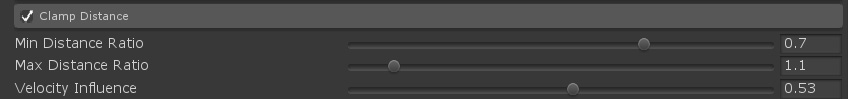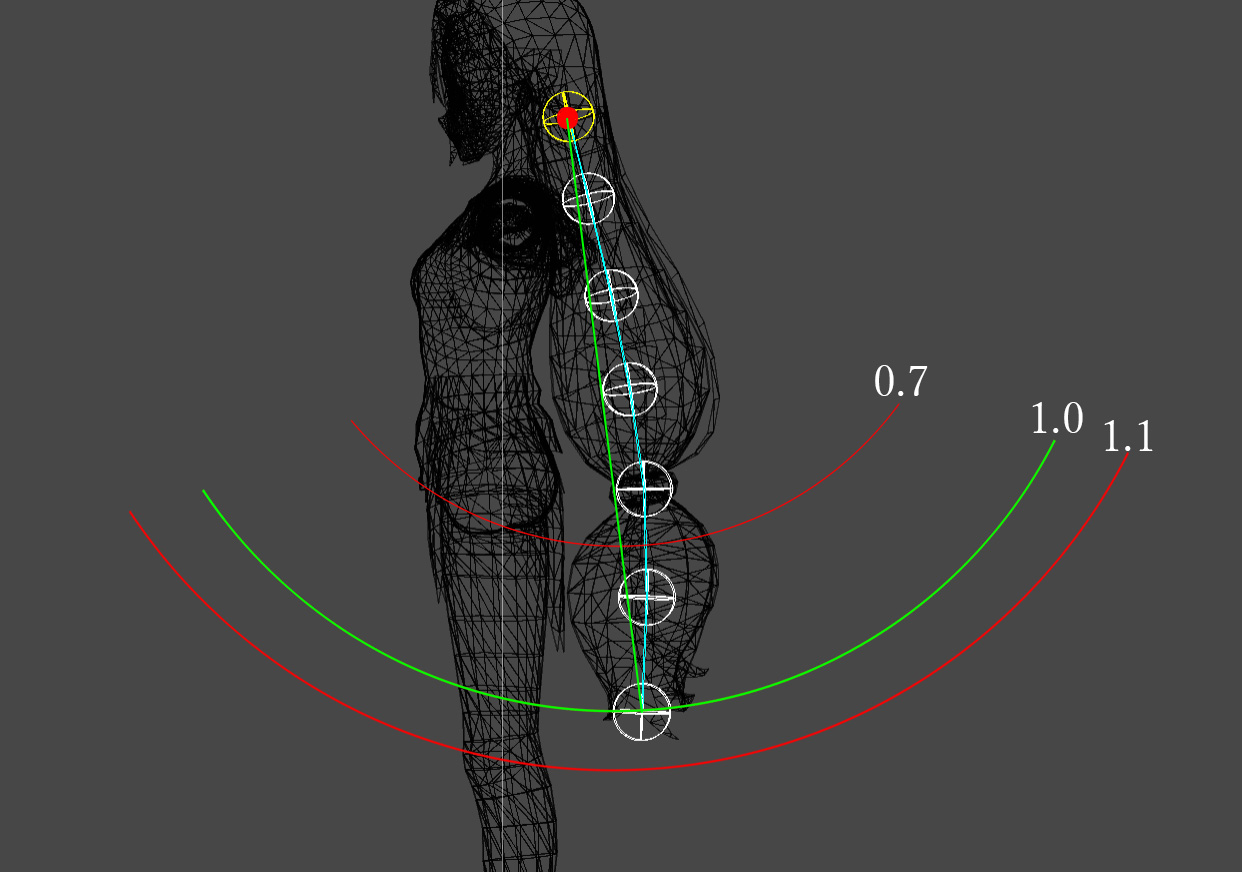# Clamp Distance

###### Maximum travel distance from start pointYou can set the maximum distance that particles can move from the starting point.
By setting this, you can avoid the phenomenon that the shape expands and contracts extremely.

As the reference distance, set the straight line distance from the starting point to the origin as 1.0, and set the extent to which the distance can be expanded and contracted as a percentage.
As an example, when [Min Distance Ratio] is 0.7 and [Max Distance Ratio] is 1.1, the movement limit range is as follows.This figure shows the movement limit range at the end (bottom) of moving particles.
The red point above is the starting point, and the green line is the distance (1.0) to the origin.
The end of moving particles can only move within the range between the red lines in the diagram.

The content of each parameter is as follows.

 Name Description Min Distance Ratio Minimum percentage of distance that can be moved from the starting point Max Distance Ratio Maximum distance that can be moved from the starting point Velocity Influence Speed effect.Setting this to a low value can reduce the problem of particles moving in response to sudden movement.However, the inertia of the particles is also lost.